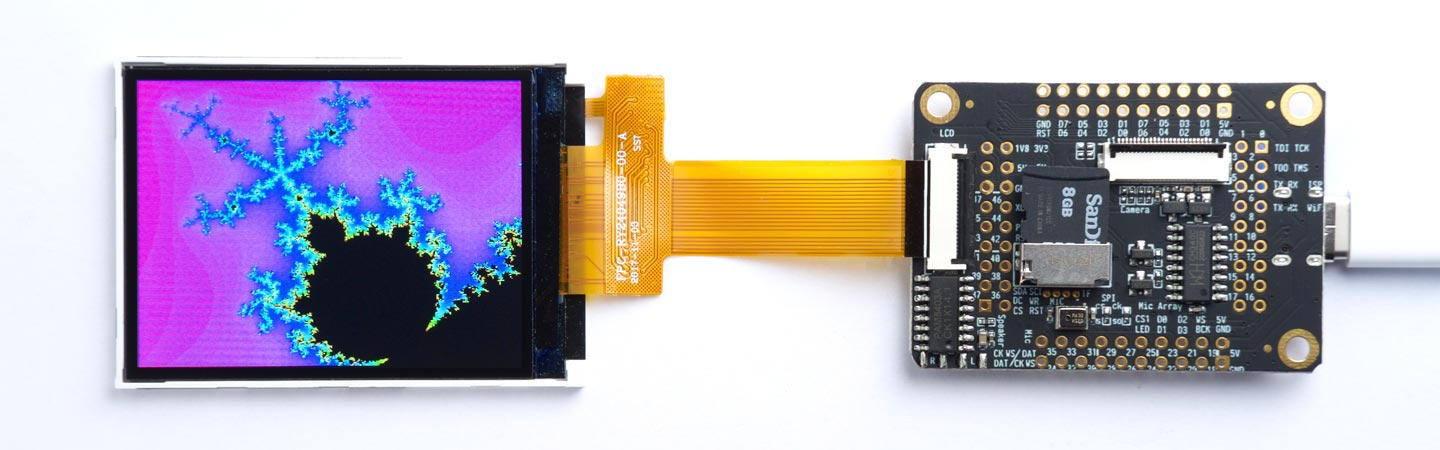## Mandelbrot set using assembler

One of the Graphics utilities is a uLisp program to plot the Mandelbrot set.

This page describes how to speed up the program by writing the inner loop in RISC-V machine code using the RISC‑V assembler.

### Mandelbrot set in uLisp

Here's the Mandelbrot set program running on a MAiX One Dock board:Here's the original program in Lisp, slightly modified to make the inner loop a separate function, iterate:

```(defun mandelbrot (x0 y0 scale)
(set-rotation 2)
(fill-screen)
(dotimes (y 240)
(let ((b (+ (/ (- y 120) 120 scale) y0)))
(dotimes (x 320)
(let* ((a (+ (/ (- x 160) 120 scale) x0))
(c (iterate a b)))
(draw-pixel x y (if (plusp c) (hsv (* 359 (/ c 80)) 1 1) 0)))))))```

Here's the iterate function:

```(defun iterate (a0 b0)
(let ((c 80) (a a0) (b b0) a2)
(loop
(setq a2 (+ (- (* a a) (* b b)) a0))
(setq b (+ (* 2 a b) b0))
(setq a a2)
(decf c)
(when (or (> (+ (* a a) (* b b)) 4) (zerop c)) (return c)))))```

These functions also call rgb and hsv to choose the colours for the contours:

```(defun rgb (r g b)
(logior (ash (logand r #xf8) 8) (ash (logand g #xfc) 3) (ash b -3)))```
```(defun hsv (h s v)
(let* ((chroma (* v s))
(x (* chroma (- 1 (abs (- (mod (/ h 60) 2) 1)))))
(m (- v chroma))
(i (truncate h 60))
(params (list chroma x 0 0 x chroma))
(r (+ m (nth i params)))
(g (+ m (nth (mod (+ i 4) 6) params)))
(b (+ m (nth (mod (+ i 2) 6) params))))
(rgb (round (* r 255)) (round (* g 255)) (round (* b 255)))))```

To plot the whole Mandelbrot set call:

`(mandelbrot -0.5 0 1)`

The section I displayed in the above photograph is obtained with:

`(mandelbrot -0.53 -0.61 11)`

For convenience, here's a function go that plots this and returns the time taken:

`(defun go () (for-millis () (mandelbrot -0.53 -0.61 11)))`

On a MAiX board running at 400 MHz the uLisp version takes 230 seconds.

### Converting the iterate function to assembler

To speed up the plotting I rewrote the iterate function in RISC-V assembler, using the assembler written in uLisp.

First load the assembler code from here: RISC-V assembler in uLisp.

Fortunately the K210 processor used on the MAiX boards includes floating-point instructions, so we can use these to perform the arithmetic. I didn't include support for these in the original assembler so they need to be added from here: RISC-V assembler floating-point extensions.

Here's the assembler version of iterate. It's pretty much a direct conversion of the uLisp version above:

```(defcode iterate (a b)
(\$flw 'fa0 8 '(a0)) ;a0
(\$flw 'fa1 8 '(a1)) ;b0
(\$fmv.s 'ft0 'fa0) ;a
(\$fmv.s 'ft1 'fa1) ;b
(\$li 'a4 2)
(\$fcvt.s.w 'ft5 'a4) ; ft5=2
(\$li 'a0 80)
again
(\$fmul.s 'ft2 'ft0 'ft0)
(\$fmul.s 'ft3 'ft1 'ft1)
(\$fsub.s 'ft4 'ft2 'ft3)
(\$fmul.s 'ft6 'ft0 'ft1)
(\$fmul.s 'ft7 'ft6 'ft5)
(\$fmv.s 'ft0 'ft4) ;a